# Stereophonic Amplitude-Panning: A Derivation of the 'Tangent Law'

In a recent Forum post here on dsprelated.com the audio signal processing subject of stereophonic amplitude-panning was discussed. And in that Forum thread the so-called "Tangent Law", the fundamental principle of stereophonic amplitude-panning, was discussed. However, none of the Forum thread participants had ever seen a derivation of the Tangent Law. This blog presents such a derivation and if this topic interests you, then please read on.

The notion of stereophonic amplitude-panning is illustrated in Figure 1. The left and right speakers, both radiating the same audio signal at different amplitudes, are located symmetrically and equidistant from the Listener. (The equidistant speaker location condition ensures that the audio from the two speakers arrives to the Listener in phase.) Speaker gains gL and gR are both scalars in the range of 0 –to- 1, and they determine the energy of the audio radiated by each speaker.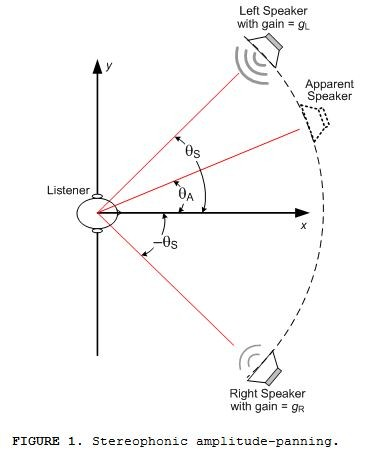When the two speakers are radiating the same amplitude audio signal (gL = gR) the Listener perceives the audio as originating from the direction aligned with the x‑axis.

Experimentation has shown that when the left Speaker is radiating a higher amplitude audio signal than the Right Speaker (gL > gR) the Listener perceives the audio as originating from the $\theta_A$ direction of the Apparent Speaker shown in Figure 1. This effect is what's called "stereophonic amplitude-panning". And in this context panning means controlling the apparent direction-of-arrival of an audio signal.

The most common expression relating Figure 1's gL and gR gain factors and the $\theta_S$ and $\theta_A$ angles is given in Eq. (1), the so-called "Tangent Law".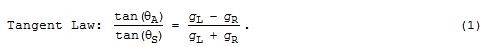Equation (1)'s Tangent Law is routinely presented in the literature of stereophonic amplitude-panning literature, but its derivation is typically missing in the literature. I spent an entire afternoon searching the web for the derivation of Eq. (1) without success. As such this blog presents my derivation the Tangent Law.

Derivation of Eq. (1)

The first step in deriving Eq. (1) is to identify three unity-magnitude two-dimensional vectors SL, SR, and A, as shown in Figure 2. Forcing them to be unit-length is important because it allows us to express their x- and y-components as simple trigonometric functions [1,2]. For example, vector SR's x- and y‑components are [cos($\theta_S$), -sin($\theta_S$)] as shown in Figure 2.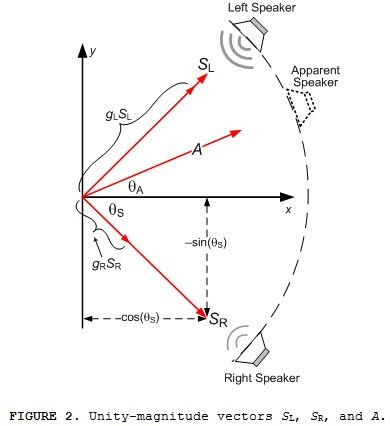Vectors SL, SR, and A. are directed toward the Left, Right, and Apparent speakers respectively.

Vector gLSL, in Figure 2, oriented in the same direction as vector SL, represents the audio signal's magnitude radiated to the Listener from the direction of the Left Speaker. Likewise, vector gRSR, oriented in the same direction as vector SR, represents the audio signal's magnitude radiated to the Listener from the direction of the Right Speaker.

Next we illustrate the x- and y-components of the SL, SR, and A vectors as shown in Figure 3.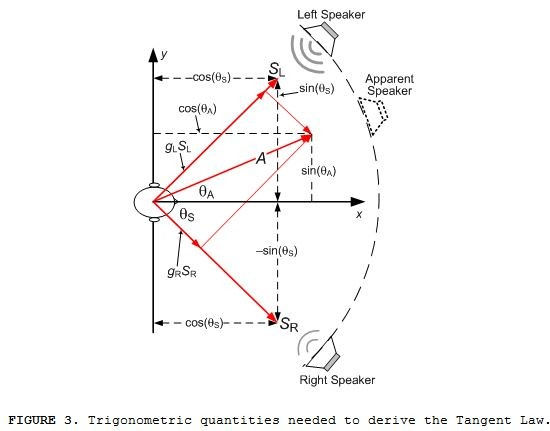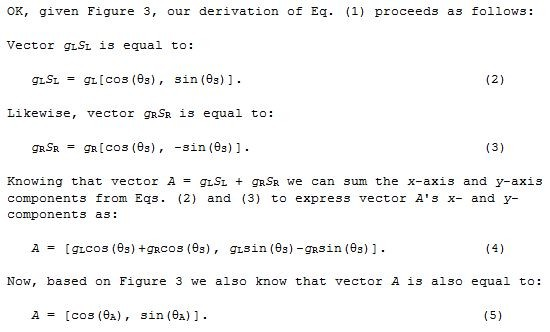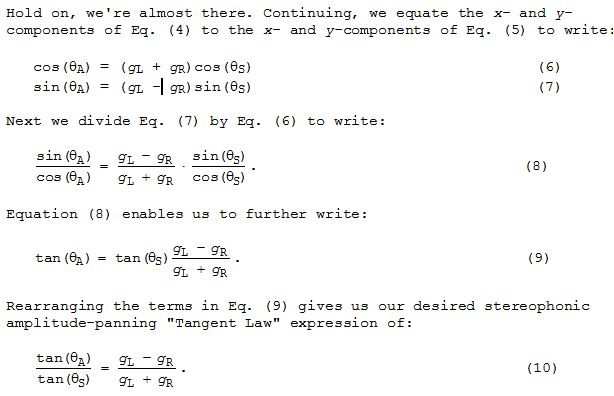Equation (10) completes our derivation of stereophonic amplitude-panning's Tangent Law.

Making the Tangent Law Useful

The "Tangent Law" expression in Eq. (10) is important but not so useful if we want to know the gL and gR gains needed to achieve a desired panning angle $\theta_A$ for a given $\theta_S$. We can determine the gL and gR gains in terms of angles $\theta_S$ and $\theta_A$ from Eqs. (6) and (7).

We start by solving Eq. (6) for gL, giving us: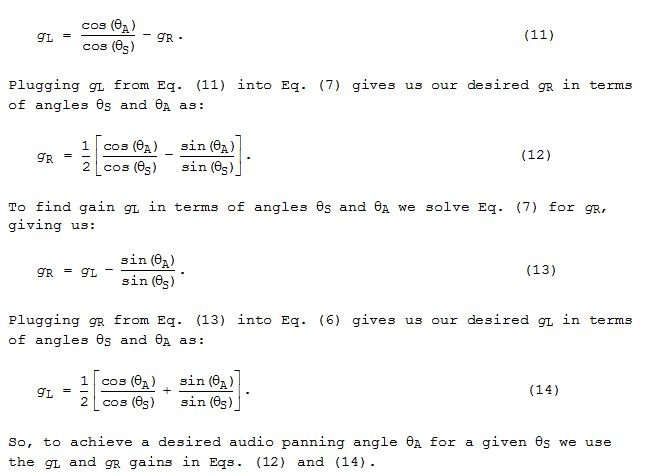So, to achieve a desired audio panning angle $\theta_A$ for a given $\theta_S$ we use the gL and gR gains in Eqs. (12) and (14).Conclusions

We presented a derivation of stereophonic amplitude-panning's Tangent Law, Eqs. (1) and (10). In addition we presented the dual gain equations needed to implement stereophonic amplitude-panning, Eqs. (12) and (14)References

 A. Blumlein, U.K. patent 394,325, 1931. Reprinted in Stereophonic Techniques (Audio Engineering Society), New York, 1986.

 B. Bauer, "Phasor Analysis of Some Stereophonic Phenomena", Journal of the Acoustical Society of America., Vol. 33, (1961 Nov.), pp. 1536-1539.

[ - ]
Comment by February 20, 2019In equation 7, shouldn't the plus sign be a minus sign?

[ - ]
Comment by February 21, 2019Hi Brian.

Yes, you're correct. Thanks for catching that typo.

[ - ]
Comment by September 14, 2020Rick - Thank you for this nice writeup - I plan to use it in my audio class.  I'm confused over the very last equation, the second part of Eqn 14 that says gL = sqrt(1-gR^2).  Shouldn't the relationship between gL and gR always depend explicitly on theta_A? Thank you!  Mark

[ - ]
Comment by September 14, 2020Hello Mark.

Good Lord! I've reviewed my Eq. (14) and for the life of me I can't figure out what I was thinking when I entered the right side of that equation. Sheece, that square root term makes no sense to me now.

Mark, I corrected my blog and I've sent a revised PDF file of this blog to Stephane. I say "Thanks a lot" to you for pointing this out to me.

[ - ]
Comment by September 16, 2020Hi Rick -  Thank you very much for looking into that.  Your writeup is great - I appreciate your taking the time to share this.  Mark

[ - ]
Comment by September 16, 2020Hi Mark.

You're most welcome. By any chance will any part of your audio class be on the Internet?

[ - ]
Comment by October 2, 2020Hi Rick -  I'm sorry for the long silence - I don't regularly check the forum. I just joined and am not in the habit yet!  I have not put any of my current class on the Internet, it's my first time on this particular course and it needs a lot of refinement!  Best, Mark

[ - ]
Comment by October 3, 2020Hi Mark.

I understand. Good Luck with your class!

To post reply to a comment, click on the 'reply' button attached to each comment. To post a new comment (not a reply to a comment) check out the 'Write a Comment' tab at the top of the comments.